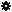﻿ Object Model: PolygonMesh.GetTriangleWeightArray

PolygonMesh.GetTriangleWeightArray

## PolygonMesh.GetTriangleWeightArrayv5.0

### Description

Returns the barycentric weight triplets describing the position within the polygon subtriangle on which the point locators are defined.

The components defining the subtriangle queried with methods PolygonMesh.GetTriangleVertexIndexArray and PolygonMesh.GetTriangleNodeIndexArray.

Notice that this information may exceptionally vary between geometry instances having the same topology but different deformations. Since the point locators are defining a precise position on the surface, and since deformations can dynamically change the triangulation within a polygon, it can happen that a point locator is remapped to a different subtriangle (both barycentric weights and subtriangle indices can vary).

An example using this method can be found in PolygonMesh.GetPolygonIndexArray and Geometry.GetClosestLocations methods' description.

### C# Syntax

 ```Object PolygonMesh.GetTriangleWeightArray( PointLocatorData in_pPtLocators, Object in_pPointLocatorsIndices ); ```

### Scripting Syntax

 ```oArray = PolygonMesh.GetTriangleWeightArray( PointLocators, [Indices] ); ```

### Return Value

2-dimensional Array of UVW triplets (barycentric coordinates)

### Parameters

Parameter Type Description
PointLocators PointLocatorData Point locators to be described.
Indices 1-dimensional Array Indices of the point locators to be described (all in not specified).